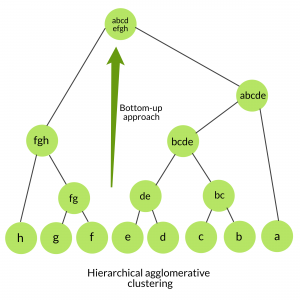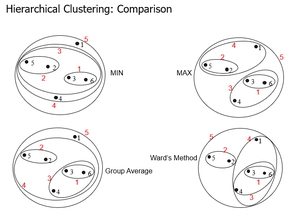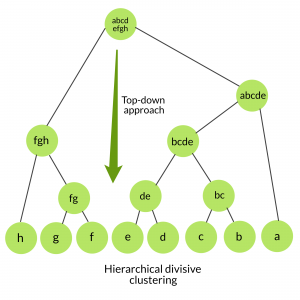Open in App
Not now

# ML | Hierarchical clustering (Agglomerative and Divisive clustering)

• Difficulty Level : Hard
• Last Updated : 19 Sep, 2022

In data mining and statistics, hierarchical clustering analysis is a method of cluster analysis that seeks to build a hierarchy of clusters i.e. tree-type structure based on the hierarchy.

Basically, there are two types of hierarchical cluster analysis strategies –

1. Agglomerative Clustering: Also known as bottom-up approach or hierarchical agglomerative clustering (HAC). A structure that is more informative than the unstructured set of clusters returned by flat clustering. This clustering algorithm does not require us to prespecify the number of clusters. Bottom-up algorithms treat each data as a singleton cluster at the outset and then successively agglomerates pairs of clusters until all clusters have been merged into a single cluster that contains all data.

Algorithm :

```given a dataset (d1, d2, d3, ....dN) of size N
# compute the distance matrix
for i=1 to N:
# as the distance matrix is symmetric about
# the primary diagonal so we compute only lower
# part of the primary diagonal
for j=1 to i:
dis_mat[i][j] = distance[di, dj]
each data point is a singleton cluster
repeat
merge the two cluster having minimum distance
update the distance matrix
until only a single cluster remains```Python implementation of the above algorithm using the scikit-learn library:

## Python3

 `from` `sklearn.cluster ``import` `AgglomerativeClustering` `import` `numpy as np`   `# randomly chosen dataset` `X ``=` `np.array([[``1``, ``2``], [``1``, ``4``], [``1``, ``0``],` `              ``[``4``, ``2``], [``4``, ``4``], [``4``, ``0``]])`   `# here we need to mention the number of clusters ` `# otherwise the result will be a single cluster` `# containing all the data` `clustering ``=` `AgglomerativeClustering(n_clusters ``=` `2``).fit(X)`   `# print the class labels` `print``(clustering.labels_)`

Output :

`[1, 1, 1, 0, 0, 0]`

Computing Distance Matrix: While merging two clusters we check the distance between two every pair of clusters and merge the pair with least distance/most similarity. But the question is how is that distance determined. There are different ways of defining Inter Cluster distance/similarity. Some of them are:

1. Min Distance: Find minimum distance between any two points of the cluster.

2. Max Distance: Find maximum distance between any two points of the cluster.

3. Group Average: Find average of distance between every two points of the clusters.

4. Ward’s Method: Similarity of two clusters is based on the increase in squared error when two clusters are merged.

For example, if we group a given data using different method, we may get different results:2. Divisive clustering: Also known as a top-down approach. This algorithm also does not require to prespecify the number of clusters. Top-down clustering requires a method for splitting a cluster that contains the whole data and proceeds by splitting clusters recursively until individual data have been split into singleton clusters.

Algorithm :

```given a dataset (d1, d2, d3, ....dN) of size N
at the top we have all data in one cluster
the cluster is split using a flat clustering method eg. K-Means etc
repeat
choose the best cluster among all the clusters to split
split that cluster by the flat clustering algorithm
until each data is in its own singleton cluster```Hierarchical Agglomerative vs Divisive clustering –

• Divisive clustering is more complex as compared to agglomerative clustering, as in the case of divisive clustering we need a flat clustering method as “subroutine” to split each cluster until we have each data having its own singleton cluster.
• Divisive clustering is more efficient if we do not generate a complete hierarchy all the way down to individual data leaves. The time complexity of a naive agglomerative clustering is O(n3) because we exhaustively scan the N x N matrix dist_mat for the lowest distance in each of N-1 iterations. Using priority queue data structure we can reduce this complexity to O(n2logn). By using some more optimizations it can be brought down to O(n2). Whereas for divisive clustering given a fixed number of top levels, using an efficient flat algorithm like K-Means, divisive algorithms are linear in the number of patterns and clusters.
• A divisive algorithm is also more accurate. Agglomerative clustering makes decisions by considering the local patterns or neighbor points without initially taking into account the global distribution of data. These early decisions cannot be undone. whereas divisive clustering takes into consideration the global distribution of data when making top-level partitioning decisions.

My Personal Notes arrow_drop_up
Related Articles